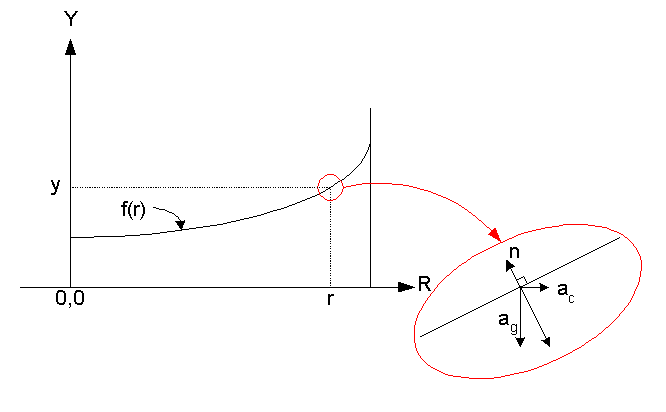# Height of the water in a rotating water pot

1. A pot of 'm' water is rotating about Y axis with w angular velocity...Water is not compressible here... What's the height of the water?

2. Fc=m ω^2 r F=mg m/v=ρ=>

3. I solved the ques for rotation around X axis... Fc =mg From that I found out the h
But then I found out that its Y axis... The less amount of data discourages me to look for solution using kutta Jowkowski theorem... I was thinking adding the two force F and Fc... Then reaction... My ques is will the reaction on the water by the water pot change the h? Pretty much confused here...

haruspex
Homework Helper
Gold Member
2020 Award
2. Fc=m ω^2
What's Fc? If it's a force the RHS is dimensionally wrong.
Edit: Just realised the following 'r' is part of the equation: Fc=m ω2r. Still like to know what Fc is.

What's Fc?

Since $\omega = v / r$,
$$m \omega^2 r = m (v/r)^2 r = m v^2 / r$$
which is the centripetal force.

Fc is the centripetal force... If it was rotating around x axis,I would just say Fc equals to the weight of the object... so that the water remains in the bucket...

haruspex
Homework Helper
Gold Member
2020 Award
I'm puzzled by the discussion about X axis and Y axis. Sounds to me like it's rotating about a vertical axis, and we're looking for how high the water level goes at the side. Is that it?

256bits
Gold Member
It appears that the question is about a pot of water rotating about its axis of symmetry.
The axis of rotation is the vertical y-axis.

Axis of symmetry is important - the pot could be rotating about a vertical axis, but some distance ( r ) from the axis at the end of an arm or having the handle attached to a rope.

256bits is right...

Yes haruspex that's it...

Now to solve the problem... I applied bernouli's theorem... But confused about my answer...

P is constant...

p g h1 = 1/2 p w^2 r^2 + p g h2

h1 - w^2 r^2 / 2g = h2

I don't feel I am right... I thought the water would be down in the middle and high in the sides... But this approach gives me lower height to the farther side of the pot... highest height in the side closer to the vertical axis...

what do u think?

what was the conclusion of that thread? What is the shape of the water surface?

gneill
Mentor
Suppose that the water has been rotating for a long time and has reached a stable equilibrium, wherein all relative motion of the water has ceased (no currents). In the rotating frame of reference we can consider the profile view of the water distribution, and let the curve representing the surface of the water be a function f(r).Now consider a small parcel of water on the surface of the water at some distance r from the axis of rotation. Since all relative motion has ceased and that parcel must be stationary, what must be the net force acting on it? More importantly, in what direction must the "weight" of the parcel be pointing? What can you then say about the slope of the curve f(r) at that point?

#### Attachments

256bits
Gold Member
P is constant...

p g h1 = 1/2 p w^2 r^2 + p g h2

h1 - w^2 r^2 / 2g = h2

I don't feel I am right... I thought the water would be down in the middle and high in the sides... But this approach gives me lower height to the farther side of the pot... highest height in the side closer to the vertical axis...

what do u think?

You are solving via energy balance, and the approach looks OK,
but you have a sign problem.

With pgh as h increases the potential energy increases due to the gravitational field.
With rotation, as r increases from the axis of rotation, the potential energy DECREASES.
so you should have
U(0) = U(r)
U(0) = pgh(0) - 1/2 p w^2 r(0)^2 ; where 1/2 p w^2 r(0)^2 = 0
U(r) = pgh(r) -1/2 p w^2 r^2

thus
pgh(0) - 0 = pgh(r) -1/2 p w^2 r^2
h(0) + 1/2g w^2 r^2 = h(r)

Note that you are using a reference frame moving along with the water, with the fictional centrifugal force providing the potential energy of an element of liquid at distance r from the axis.

See if you can get the same answer, from the post of gneill, using forces in a co-moving reference frame.

Thank you very much for your help guys... :)May I ask what you used to create this diagram? Thanks.

gneill
Mentor
May I ask what you used to create this diagram? Thanks.

Sure. Microsoft Visio 2000.